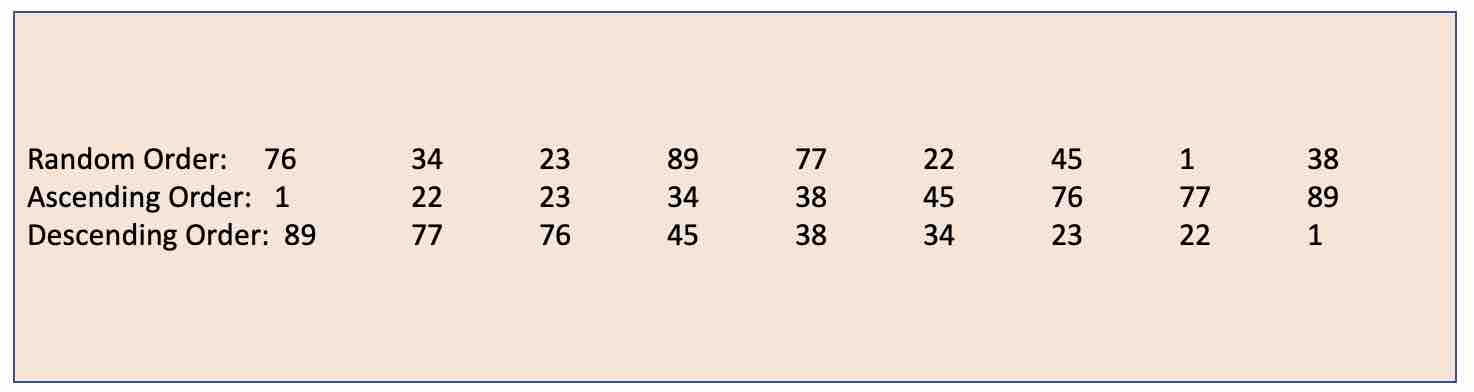# Sort Array in Ascending or Descending Order in JavaIn this program, we will take a look at how you can sort an Array in Java in Ascending or Descending order

First let's create an array with random numbers.

``int[] randomNumberArray = {76,34,23,89,77,22,45,1,38};``

## Sorting in Ascending Order

Now to sort this array of numbers in ascending order we can make use of the Arrays.sort method.

``Arrays.sort(randomNumberArray);``

Finally let's iterate through the array and print the sorted array values

``````for (int no: randomNumberArray) {
System.out.print(no +"\t");
}``````

1 22 23 34 38 45 76 77 89

## Sorting in Descending Order

Note that there is no revere function in the Arrays utility class to reverse an Array and make it in descending order. So we have to either use Collections class or write our own logic.

``````    public static void main(String[] args) {

int[] randomNumberArray = {76,34,23,89,77,22,45,1,38};

Arrays.sort(randomNumberArray);

// Reverse the array
for (int i = 0; i < randomNumberArray.length / 2; i++) {
int temp = randomNumberArray[i];
randomNumberArray[i] = randomNumberArray[randomNumberArray.length - i - 1];
randomNumberArray[randomNumberArray.length - i - 1] = temp;
}

for (int no: randomNumberArray) {
System.out.print(no +"\t");
}
}``````

89 77 76 45 38 34 23 22 1

-

Have Questions? Post them here!